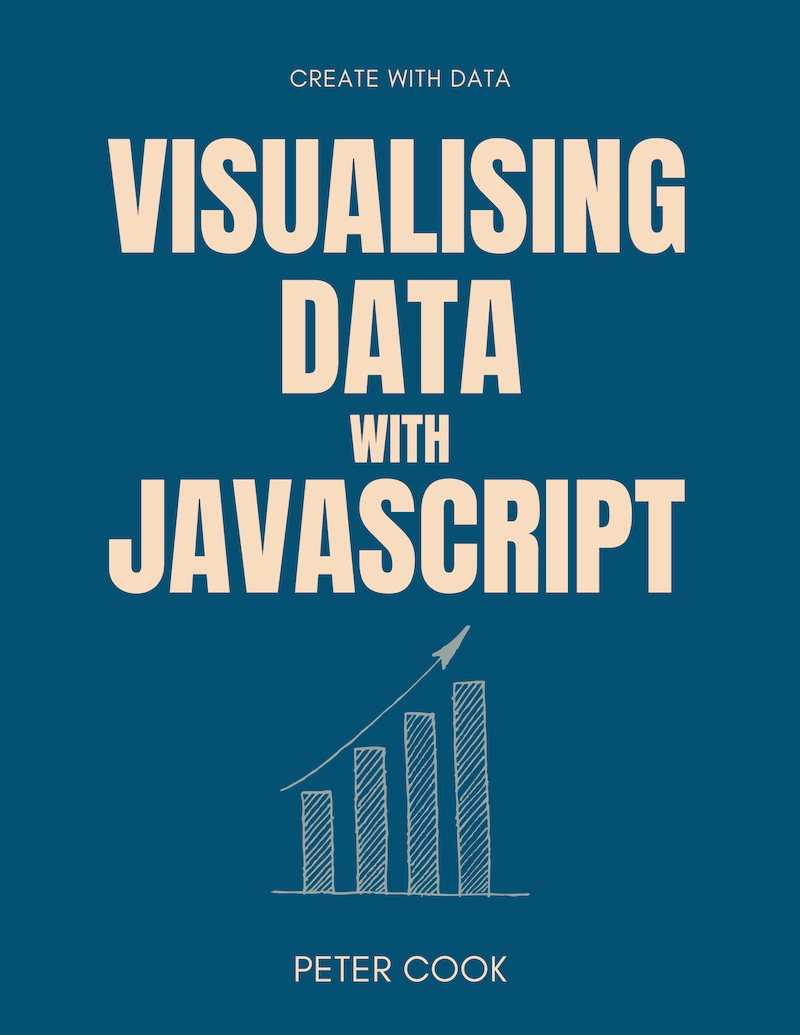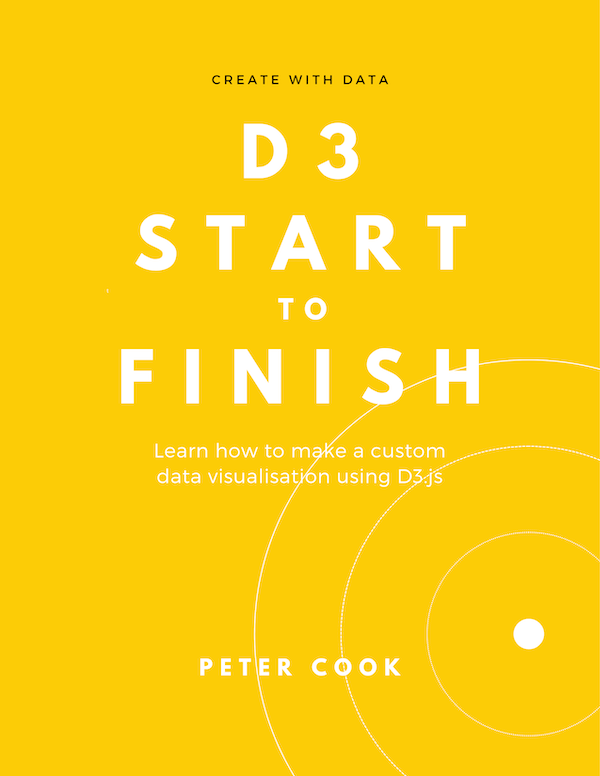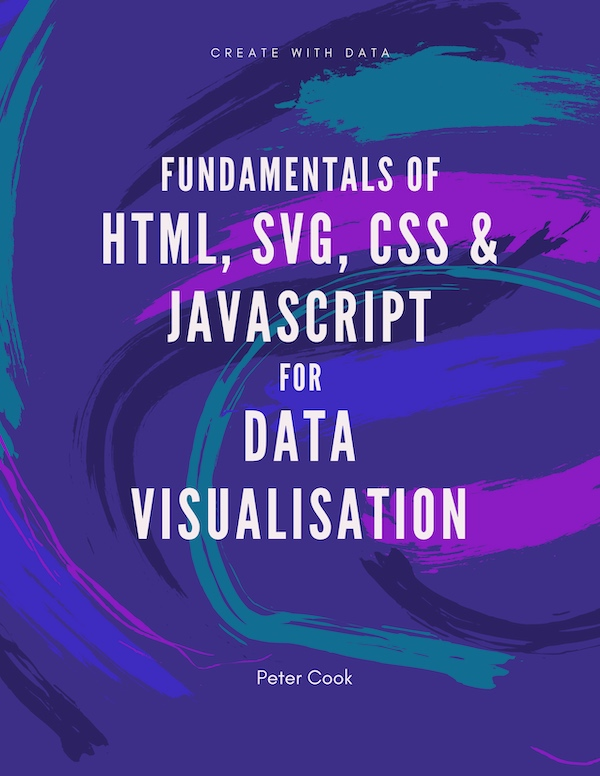D3 in Depth covers versions 6 and 7 of D3

Get book discounts and receive D3.js related news and tips.

Subscribe

# D3 Data Joins

This article shows how to join an array of data to a D3 selection. We cover how to create a data join, how to update a data join, how to join an array of objects and key functions. We also show how to simplify your data join code using layout functions.

A data join creates a correspondence between an array of data and a selection of HTML or SVG elements.

Joining an array to HTML/SVG elements means that:

• HTML (or SVG) elements are added or removed such that each array element has a corresponding HTML (or SVG) element
• each HTML/SVG element may be positioned, sized and styled according to the value of its corresponding array element

For example, suppose you've an array of five numbers which you'd like to join to `circle` elements:

``[ 40, 10, 20, 60, 30 ]``

Each time D3 performs the join it'll add or remove `circle` elements so that each array element has a corresponding `circle` element.

D3 can also update the position and radius of each circle (and any other attributes or style) based on the value of the corresponding array element.

For example, the radius of each circle can be set to half the value of the corresponding array value. This results in the first circle having a radius of 20, the second a radius of 5 and so on:

Prior to version 5 of D3, data joins were not all that easy to learn (you had to learn about enter, exit and update). Fortunately, for versions 5 and up, data joins are much easier!

## How to create a data join

The general pattern for creating a data join is:

``d3.select(container)  .selectAll(element-type)  .data(array)  .join(element-type);``

where:

• `container` is a CSS selector string that specifies a single element that'll contain the joined HTML/SVG elements
• `element-type` is a string describing the type of element you’re joining (e.g. ‘div' or 'circle')
• `array` is the name of the array you’re joining

Typically four methods are used in a data join:

• `.select` defines the element that'll act as a container (or parent) to the joined HTML/SVG elements
• `.selectAll` defines the type of element that'll be joined to each array element
• `.data` defines the array that's being joined
• `.join` performs the join. This is where HTML or SVG elements are added and removed

### Example

Given an array:

``let myData = [40, 10, 20, 60, 30];``

and an `svg` element containing a `g` element:

``<svg>  <g class="chart">  </g></svg>``

you can join `myData` to `circle` elements using:

``let myData = [40, 10, 20, 60, 30];d3.select('.chart')  .selectAll('circle')  .data(myData)  .join('circle');``

In this example:

• the container is the `g` element
• the element type is `circle`
• the array being joined is `myData`

Running this code results in 5 circles being created:

``<svg>  <g class="chart">    <circle></circle>    <circle></circle>    <circle></circle>    <circle></circle>    <circle></circle>  </g></svg>``

You can't see any circles because each radius is zero. However if you inspect and expand the SVG element (right click on the SVG element which has been coloured light pink and select Inspect) you'll see five `circle` elements have been added:In the CodePen example you can try adding elements to the array and you'll see that the data join ensures there are as many circles as array elements.

### Updating the joined elements

Joined HTML or SVG elements may be updated using the `.style`, `.attr` and `.text` methods that were covered in the selections chapter. (The `.join` method returns a selection containing all of the joined elements.)

For example you can set the center, radius and colour of every circle using:

``let myData = [40, 10, 20, 60, 30];d3.select('.chart')  .selectAll('circle')  .data(myData)  .join('circle')  .attr('cx', 200)  .attr('cy', 50)  .attr('r', 40)  .style('fill', 'orange');``

You only see one circle because all five circles share the same position and size.

If a function is passed into `.attr` or `.style` you can update the HTML/SVG elements in a data-driven manner.

The function is called for each element in the selection. It takes two parameters, typically named `d` and `i`.

The first parameter (`d`) represents the corresponding array element (or the 'joined value'). The second parameter `i` represents the index of the element within the selection.

The return value of the function is used to set the attribute or style value.

Let's pass a function into the first `.attr`:

``let myData = [40, 10, 20, 60, 30];d3.select('.chart')  .selectAll('circle')  .data(myData)  .join('circle')  .attr('cx', function(d, i) {    return i * 100;  })  .attr('cy', 50)  .attr('r', 40)  .style('fill', 'orange');``

This tells D3 to set each circle's `cx` attribute to `i * 100`. `i` is the index within the selection, so the first circle will be positioned at `0`, the next at `100` and so on:

Now let's set `r` according to the joined value:

``let myData = [40, 10, 20, 60, 30];d3.select('.chart')  .selectAll('circle')  .data(myData)  .join('circle')  .attr('cx', function(d, i) {    return i * 100;  })  .attr('cy', 50)  .attr('r', function(d) {    return 0.5 * d;  })  .style('fill', 'orange');``

(The first circle is clipped because it's `cx` attribute is zero.)

You can put any amount of logic within the functions that are passed into `.attr` and `.style`. For example, let's colour the circle if its joined value is greater than 30:

``let myData = [40, 10, 20, 60, 30];d3.select('.chart')  .selectAll('circle')  .data(myData)  .join('circle')  .attr('cx', function(d, i) {    return i * 100;  })  .attr('cy', 50)  .attr('r', function(d) {    return 0.5 * d;  })  .style('fill', function(d) {    return d > 30 ? 'orange' : '#eee';  });``

The `.join` method returns a D3 selection, so you can use any of the methods covered in the selections chapter such as `.style`, `.attr`, `.text` and `.each`

### Joining arrays of objects

When building data visualisations with D3 you'll typically be joining arrays of objects (rather than arrays of numbers). For example:

``let cities = [  { name: 'London', population: 8674000},  { name: 'New York', population: 8406000},  { name: 'Sydney', population: 4293000},  { name: 'Paris', population: 2244000},  { name: 'Beijing', population: 11510000}];``

You can join an array of objects in the same manner as before. However, when a function is passed into `.attr` or `.style`, the `d` parameter is an object. This means that you'll typically reference a property of that object.

For example:

``  ...  .attr('r', function(d) {    return 0.0001 * d.population;  })  ...``

Here's a full example where `cities` is joined to `circle` elements:

``var cities = [  { name: 'London', population: 8674000},  { name: 'New York', population: 8406000},  { name: 'Sydney', population: 4293000},  { name: 'Paris', population: 2244000},  { name: 'Beijing', population: 11510000}];d3.select('.chart')  .selectAll('circle')  .data(cities)  .join('circle')  .attr('cx', function(d, i) {    return i * 100;  })  .attr('cy', 50)  .attr('r', function(d) {    let scaleFactor = 0.00004;    return scaleFactor * d.population;  })  .style('fill', '#aaa');``

Each circle is sized according to the city's population. (Note that it's better practice to set the area, rather than the radius, of a circle proportionally to a data value.)

You can build a simple bar chart using the above techniques. Instead of joining `circle` elements, let's join `rect` and `text` elements:

``let cities = [  { name: 'London', population: 8674000},  { name: 'New York', population: 8406000},  { name: 'Sydney', population: 4293000},  { name: 'Paris', population: 2244000},  { name: 'Beijing', population: 11510000}];// Join cities to rect elements and modify height, width and positiond3.select('.bars')  .selectAll('rect')  .data(cities)  .join('rect')  .attr('height', 19)  .attr('width', function(d) {    let scaleFactor = 0.00004;    return d.population * scaleFactor;  })  .attr('y', function(d, i) {    return i * 20;  })// Join cities to text elements and modify content and positiond3.select('.labels')  .selectAll('text')  .data(cities)  .join('text')  .attr('y', function(d, i) {    return i * 20 + 13;  })  .text(function(d) {    return d.name;  });``

How about that? We've just built a simple bar chart using D3!

(This example relies on extra code in `index.html` and `style.css`. Open the CodePen example to see the full example.)

## Update functions

If your data array changes you'll need to perform the join again. (Unlike some frameworks such as Vue.js, D3 doesn't automatically do this for you.)

Therefore we usually put the join code in a function. Whenever the data changes, we'll call this function.

I usually name the function `update`. For example:

``let myData = [40, 10, 20, 60, 30];function update(data) {  d3.select('.chart')    .selectAll('circle')    .data(data)    .join('circle')    .attr('cx', function(d, i) {      return i * 100;    })    .attr('cy', 50)    .attr('r', function(d) {      return 0.5 * d;    })    .style('fill', function(d) {      return d > 30 ? 'orange' : '#eee';    });}update(myData);``

We pass the data array into `update`. Each time `update` is called the join is performed.

The data doesn't ever change in the previous example, so let's add a button that when clicked gets some randomised data and calls `update`:

``function getData() {  let data = [];  let numItems = Math.ceil(Math.random() * 5);  for(let i=0; i<numItems; i++) {    data.push(Math.random() * 60);  }  return data;}function update(data) {  d3.select('.chart')    .selectAll('circle')    .data(data)    .join('circle')    .attr('cx', function(d, i) {      return i * 100;    })    .attr('cy', 50)    .attr('r', function(d) {      return 0.5 * d;    })    .style('fill', function(d) {      return d > 30 ? 'orange' : '#eee';    });}function updateAll() {  let myData = getData();  update(myData);}updateAll();d3.select("button")  .on("click", updateAll);``

`getData` returns an array containing a random number of random values. A `button` element has been added to `index.html` (see the CodePen example). The last two lines add an event handler `updateAll` to this button. `updateAll` calls `getData` and then calls `update` to perform the join.

Therefore each time the button is clicked, the data updates and the circles update accordingly.

### Key functions

When D3 performs a data join it joins the first array element to the first element in the selection, the second array element to the second element in the selection and so on.

However, if the order of array elements changes (due to sorting, inserting or removing elements) the array elements can get joined to different DOM elements.

You can ensure each array element remains joined to the same HTML/SVG element by passing a key function into the `.data` method. The key function should return a unique id value for each array element.

Look at this example where an array `['Z']` is joined to `div` elements. Each time the button is clicked a new letter is added to the start of the array:

When 'Insert element' is clicked a new `div` element is added to the end of the row. However, the text of each existing `div` changes, resulting in a rather strange effect.

If a key function is used, each letter will stay joined to the same `div` element:

In general, if your array elements can change position within the array you should probably use a key function.

### Debugging

When D3 performs a data join it adds an attribute `data` to each DOM element in the selection and assigns the joined data to it.

We can inspect this in Google Chrome by right clicking on an element, choosing 'Inspect' and typing:

``\$0.__data__``

in the debug console. (`\$0` represents the element that's being inspected.)Being able to check the joined data in this manner is particularly useful when debugging as it allows us to check whether our data join is behaving as expected.

BOOKS & COURSESVisualising Data with JavaScript teaches you how to build charts, dashboards and data stories using Chart.js, Leaflet, D3 and React.

Find out more"One of the best D3 books I've read. The contents are very clear, it is easy to follow and the concepts are very solid."

Javier García Fernández

Learn how to make a custom data visualisation using D3.js.

Find out moreLearn the fundamentals of HTML, SVG, CSS and JavaScript for building data visualisations on the web.

Find out more